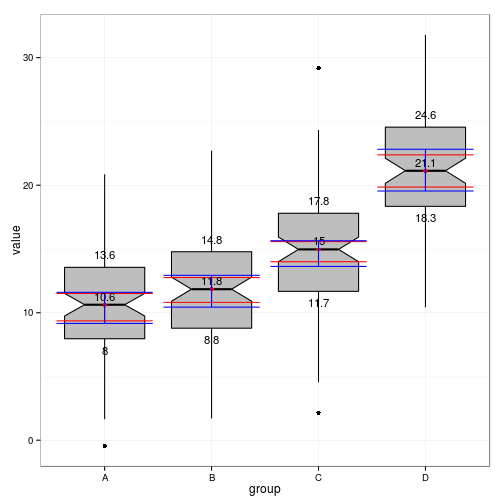# Bootstrap confidence intervals for the median

Grammar of graphics plots provide a simple way of adding confidence intervals for the mean to any boxplot. Let's set up some grouped data.

``````d <- data.frame(group = rep(c("A", "B", "C", "D"), each = 100), mean = rep(c(10,
12, 15, 20), each = 100))
d\$value <- d\$mean + rnorm(400, 0, 5)
``````

## Standard box plot

``````library(ggplot2)
library(boot)
g0 <- ggplot(d, aes(x = group, y = value))
g_box <- g0 + geom_boxplot(fill = "grey", colour = "black") + theme_bw()
g_box
``````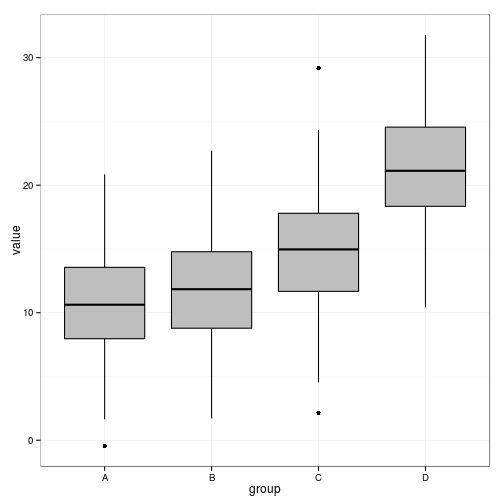## Boxplot with confidence intervals for the mean added.

``````g_box <- g_box + stat_summary(fun.data = mean_cl_boot, geom = "errorbar", colour = "red") +
stat_summary(fun.y = mean, geom = "point", colour = "red")
g_box
``````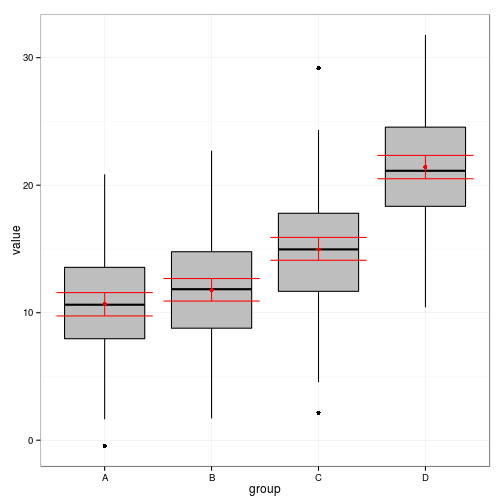It is possible to add some text to display the value of the mean.

``````g_box <- g_box + stat_summary(aes(label = round(..y.., 1)), fun.y = mean, geom = "text",
size = 6, col = "red")
g_box
``````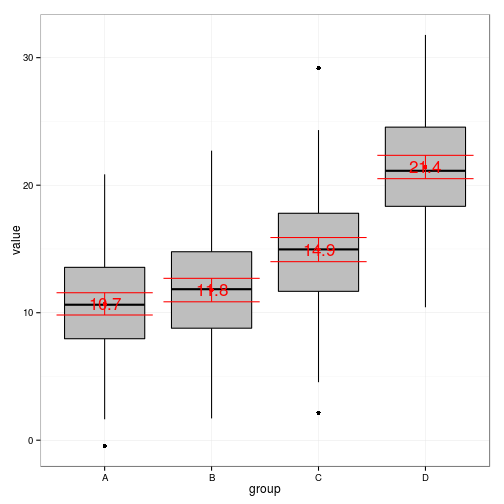``````g_box <- g_box + stat_summary(aes(label = round(..y.., 1)), fun.y = function(x) quantile(x,
0.75), geom = "text", size = 4, vjust = -1)
g_box <- g_box + stat_summary(aes(label = round(..y.., 1)), fun.y = function(x) quantile(x,
0.25), geom = "text", size = 4, vjust = 2)
g_box
``````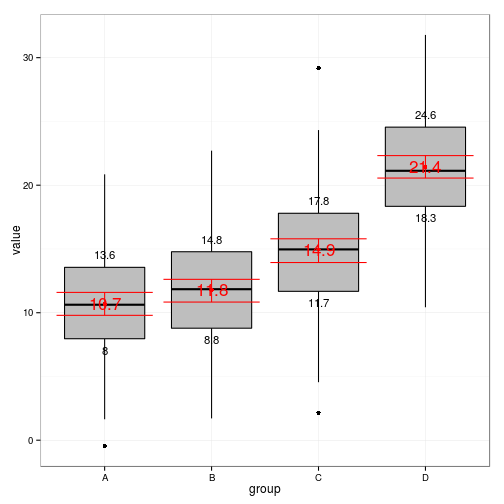## Confidence intervals for the median

One way of adding approximate confidence intervals is to use notch=TRUE.

``````g_box <- g0 + geom_boxplot(fill = "grey", colour = "black", notch = TRUE) +
theme_bw()
g_box
``````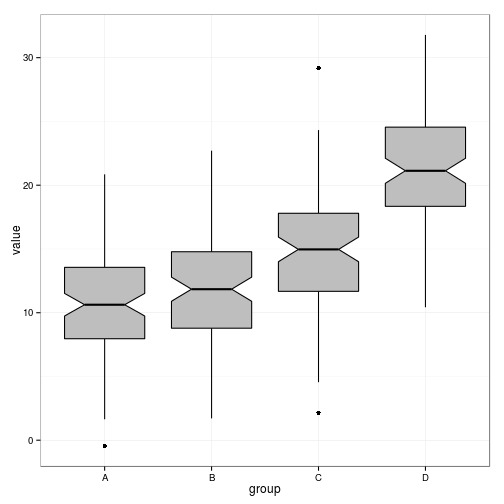### Bootstrapping the median

We need a function that returns a data frame in the same format as mean_cl_boot.

``````median_cl_boot <- function(x, conf = 0.95) {
lconf <- (1 - conf)/2
uconf <- 1 - lconf
require(boot)
bmedian <- function(x, ind) median(x[ind])
bt <- boot(x, bmedian, 1000)
bb <- boot.ci(bt, type = "perc")
data.frame(y = median(x), ymin = quantile(bt\$t, lconf), ymax = quantile(bt\$t,
uconf))
}
``````

Now we can add the bootstrapped confidence intervals for the median in the same way.

``````g_box <- g_box + stat_summary(fun.data = median_cl_boot, geom = "errorbar",
colour = "red") + stat_summary(fun.y = median, geom = "point", colour = "red")
g_box
``````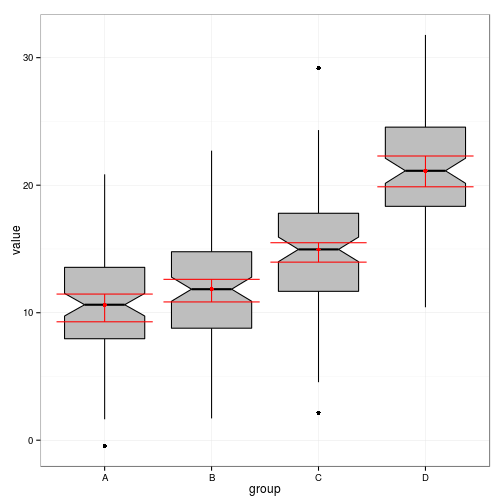The values for the median can also be added as text.

``````g_box <- g_box + stat_summary(aes(label = round(..y.., 1)), fun.y = median,
geom = "text", size = 4, vjust = -0.5)
g_box <- g_box + stat_summary(aes(label = round(..y.., 1)), fun.y = function(x) quantile(x,
0.75), geom = "text", size = 4, vjust = -1)
g_box <- g_box + stat_summary(aes(label = round(..y.., 1)), fun.y = function(x) quantile(x,
0.25), geom = "text", size = 4, vjust = 2)
g_box
``````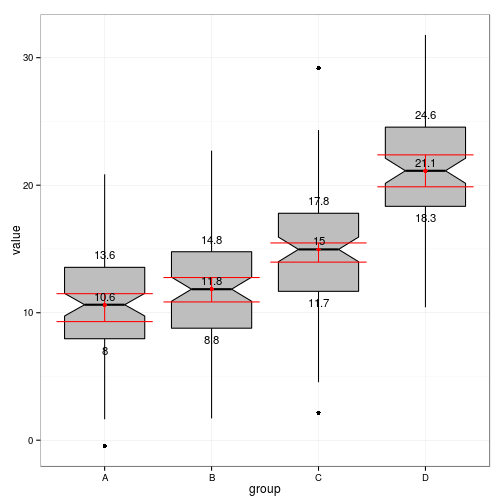Changing the confidence interval.

``````g_box + stat_summary(fun.data = median_cl_boot, conf = 0.99, geom = "errorbar",
colour = "blue")
``````